Vectors and conics

This free course is available to start right now. Review the full course description and key learning outcomes and create an account and enrol if you want a free statement of participation.

Free course

1.4 Intersection of two lines

Two arbitrary lines in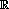2 may have a single point of intersection, may be parallel, or may coincide. The first two possibilities are illustrated below. Can we tell from the equations of the lines which of the three possibilities occurs?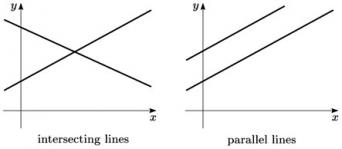We deal with this question by considering some examples. Given the equations of two lines, it is often useful to sketch the lines, since this will not only show whether the lines do intersect, but it will give us some idea of the location of any point of intersection. Then, since the coordinates of the point of intersection must satisfy the equations of both lines, we can find these coordinates by solving the two equations as simultaneous equations in x and y.

Example 2

Determine whether the following pairs of lines intersect each other at a single point, are parallel, or coincide. If the lines intersect at a single point, find its coordinates.

• (a) y = x + 1 and y = 3x − 1.

• (b) y = 2x − 2 and y = 2x + 1.

• (c) y =x − 2 and 2y = x − 4.

• (a) These lines have gradients 1 and 3, and y-intercepts 1 and −1, respectively; this enables us to sketch the lines, as shown below. Clearly the lines intersect, at some point in the right half-plane.

•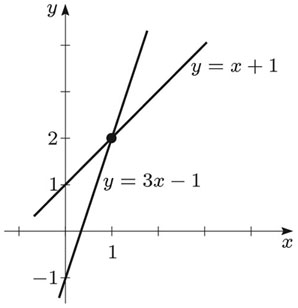• At the point of intersection, y = x + 1 and y = 3x − 1; thus x + 1 = 3x − 1, from which we deduce that x = 1.

Substituting this value of x into either equation gives y = 2. So the point of intersection of the two lines is (1, 2). (You can always check your solution of simultaneous equations by substituting your answer back into the original equations.)

• (b) These lines both have gradient 2, and their y-intercepts are −2 and 1, respectively. We can thus sketch the lines, as shown below.

•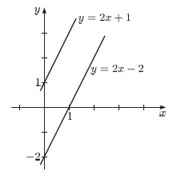• Since the lines have equal gradient and their y-intercepts are different, they are parallel and so do not intersect. (If we try to solve the equations y = 2x − 2, y = 2x + 1 as a pair of simultaneous equations, we are led to the contradiction that −2 = 1. Thus there can be no point (x, y) that lies on both lines.)

• (c) At first glance these two equations may appear to be different. However, if the second equation is divided throughout by 2 (which leaves unchanged the actual line that it represents), we obtain the first equation. So the two equations are equivalent: they represent the same line. (In a sense, the two lines intersect at each of their points.)

• The line has gradientand y-intercept −2, so we can sketch it as shown below. (Two lines coincide if the equation of one can be rearranged to be a multiple of the equation of the other.)

•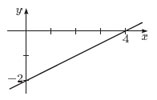Example 4

Determine whether the following pairs of lines intersect each other at a single point, are parallel, or coincide. If the lines intersect at a single point, find its coordinates.

• (a)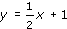and y = 2x − 1.

• (b) y = 2x − 1 and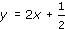.

• (c) 4y = 2x + 6 and 6y = 3x + 9.

• (d) y = 7x − 1 and y = −x.

• (a) These lines have gradientsand 2, and y-intercepts 1 and −1, respectively. This enables us to sketch the lines below. They intersect at a single point, in the right half-plane.

•

•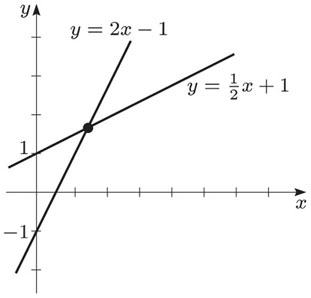• At the point of intersection,

•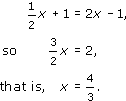• Substituting for x in the equation of the second line, we obtain

•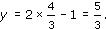• Thus the point of intersection of the lines is

•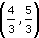• (b) These lines both have gradient 2. However, since they have different y-intercepts, −1 and, they do not coincide and so they are parallel.

•

•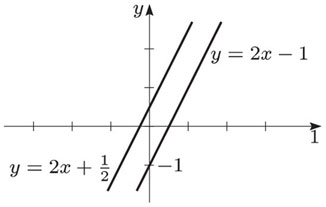• (c) These two lines coincide, since the equation of the second line is the equation of the first multiplied by.

•

•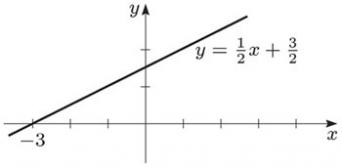• (d) These lines have gradients 7 and −1, and y-intercepts −1 and 0, respectively. This enables us to sketch the lines. They intersect at a single point, in the right half-plane.

•

•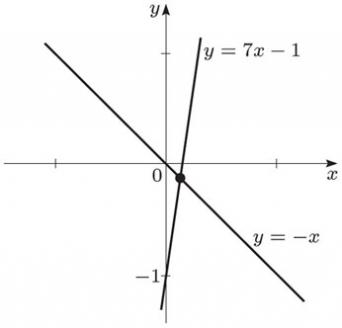• At the point of intersection

•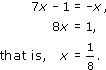• Substituting for x in the equation of the second line,

•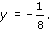• Thus the point of intersection of the lines is

•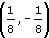M208_1